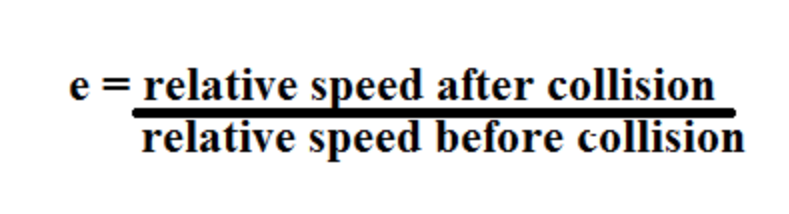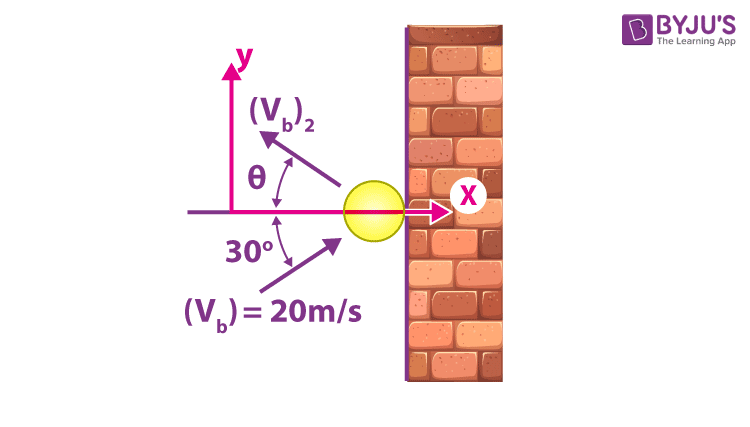# Coefficient of Restitution

## What is the Coefficient of Restitution?

The ratio of final velocity to the initial velocity between two objects after their collision is known as the coefficient of restitution. The restitution coefficient is denoted as ‘e’ and is a unitless quantity, and its values range between 0 and 1.

### A Simple Explanation of Coefficient of Restitution

Sir Issac Newton derived many mathematical equations and laws that help us make sense of what happens when two objects collide. One such important law, Newton’s law of restitution, states that when two objects collide, their speeds after the collision depend on the material they are made of.

The measure of the colliding materials’ nature is represented by a number known as the coefficient of restitution. The coefficient of restitution provides us with information about the elasticity of the collision. Collisions in which there is no loss of overall kinetic energy is known as a perfectly elastic collision. This type of collision has the maximum coefficient of restitution of e = 1. Collision, where maximum kinetic energy is lost, is known as a perfectly inelastic collision. They have a coefficient of restitution of e = 0. Most real-life collisions are in between.

### Coefficient of Restitution – Video Lesson#### Coefficient of Restitution Formula

The mathematical formula of the Coefficient of Restitution is given as follows:From the above equation, you notice that you always divide the smaller number by a more significant number. Therefore, the coefficient of restitution is always positive.

The value is almost always less than one due to initial translational kinetic energy being transformed to rotational kinetic energy, plastic deformation, and heat. However, it can be more than one if there is an energy gain during the collision from a chemical reaction, a reduction in rotational energy, or another internal energy decrease that contributes to the post-collision velocity.

 Range of Values for e If e = 0, then it is a perfectly inelastic collision If 0 < e < 1, then it is a real-world inelastic collision, in which some kinetic energy is dissipated. If e = 1, then it is a perfectly elastic collision in which no kinetic energy is dissipated, and the objects rebound from one another with the same relative speed with which they approached.

## Coefficient of Restitution in Sports

The Coefficient of Restitution plays a vital role in the design of sports balls. A basketball, for example, bounces more than a tennis ball because less energy is lost by the basketball when it hits the ground. We can determine the percentage of speed that the ball retains after the collision by use of the coefficient of restitution. The larger the value of the Coefficient of Restitution of the ball, the more elastic the collision. Furthermore, if we drop a ball from a certain height, balls with a higher Coefficient of Restitution will rebound higher.

## Coefficient of Restitution Example Problems

A ball strikes a smooth wall with a velocity (vb)1 = 20 m/s. The coefficient of restitution between the ball and the wall is e = 0.75. Find the velocity of the wall just after the impact.Solution:

The collision is an oblique impact, with the line of impact perpendicular to the plane. Thus, the coefficient of restitution applies perpendicular to the wall and the momentum of the ball is conserved along the wall.

The given problem is solved by defining x-y axes along the line perpendicular to the line of impact respectively.

The momentum of the ball is conserved in the y-direction:

$m({v_{b}}_1)\sin 30=m({v_{b}}_1)\sin\Theta$

Solving, we get

$({V_{b}}_2)\sin \Theta = 10\, m/s$……….(1)

The coefficient of restitution applies in the x-direction:

$e=\frac{[0-{(V_{bx})}_2]}{{[(V_{bx})}_1-0]}$

Solving the above equation, we get:

$(v_{b})_2\cos \Theta =12.99\, m/s$……….(2)

Solving equations (1) and (2), we get

$(v_{b})_2=(12.99^{2}+10^{2})^{0.5}=16.4\,m/s$ $\Theta =37.6^{\circ}$

## Frequently Asked Questions – FAQs

### What is the Coefficient of Restitution?

The ratio of final velocity to the initial velocity between two objects after their collision is known as the coefficient of restitution.

### What does the Coefficient of Restitution measure?

The coefficient of restitution measures the elasticity of the collision.

### How does the Coefficient of Restitution affect the collision?

The coefficient of restitution is important in collision because it determines whether a collision is elastic or inelastic.

### When is the Coefficient of Restitution zero?

When the collision is perfectly inelastic, the coefficient of restitution is zero.

### Can the coefficient of restitution be negative?

No, the coefficient of restitution is always positive.

### How does the coefficient of restitution relate to sports?

The larger the value of the Coefficient of Restitution of the ball, the more elastic the collision. Furthermore, if we drop a ball from a certain height, balls with a higher Coefficient of Restitution will rebound higher.